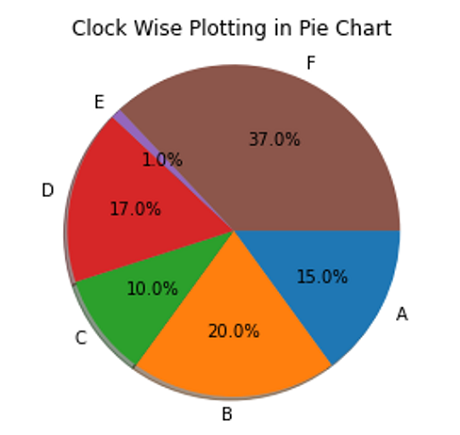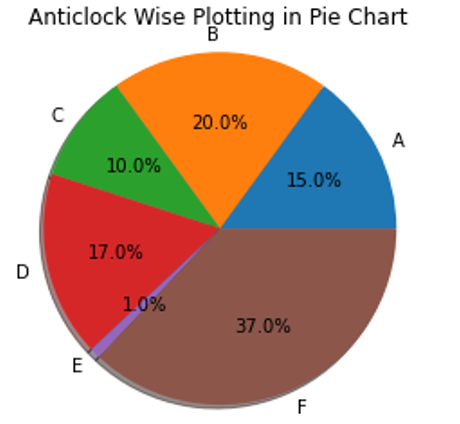# Python | Clockwise and Anticlockwise Pie Chart

In this tutorial, we are going to explore the clockwise and anticlockwise mode of plotting Pie charts.
Submitted by Anuj Singh, on August 05, 2020

Following example is an illustration for the same.

Illustration:## Python code for clockwise and anticlockwise pie chart

```import matplotlib.pyplot as plt

# Pie chart, where the slices will be
# ordered and plotted counter-clockwise:
labels = 'A', 'B', 'C', 'D', 'E', 'F'
sizes = [15, 20, 10, 17, 1, 37]
alp = (0.9, 0.9, 0.9, 0, 0.9, 0.9)
plt.figure()
plt.title('Clock Wise Plotting in Pie Chart')
plt.axis('equal')
plt.show()

plt.figure()
plt.title('Anticlock Wise Plotting in Pie Chart')
plt.axis('equal')
plt.show()
```

Output:

```Output is as figure
```

Languages: » C » C++ » C++ STL » Java » Data Structure » C#.Net » Android » Kotlin » SQL
Web Technologies: » PHP » Python » JavaScript » CSS » Ajax » Node.js » Web programming/HTML
Solved programs: » C » C++ » DS » Java » C#
Aptitude que. & ans.: » C » C++ » Java » DBMS
Interview que. & ans.: » C » Embedded C » Java » SEO » HR
CS Subjects: » CS Basics » O.S. » Networks » DBMS » Embedded Systems » Cloud Computing
» Machine learning » CS Organizations » Linux » DOS
More: » Articles » Puzzles » News/Updates